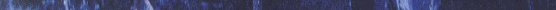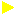# MERGE SORT## The mergesort algorithm is based on the classical divide-and-conquer paradigm. It operates as follows: DIVIDE: Partition the n-element sequence to be sorted into two subsequences of n/2 elements each. CONQUER: Sort the two subsequences recursively using the mergesort. COMBINE: Merge the two sorted sorted subsequences of size n/2 each to produce the sorted sequence consisting of n elements. Note that recursion "bottoms out" when the sequence to be sorted is of unit length. Since every sequence of length 1 is in sorted order, no further recursive call is necessary. The key operation of the mergesort algorithm is the merging of the two sorted sequencesin the "combine step". To perform the merging, we use an auxilliary procedure Merge(A,p,q,r), where A is an array and p,q and r are indices numbering elements of the array such that dure assumes that the subarrays A[p..q] and A[q+1...r] are in sorted order.It merges them to form a single sorted subarray that replaces the current subarray A[p..r]. Thus finally,we obtain the sorted array A[1..n], which is the solution.ANIMATIONTHE ALGORITHMApplet page